## 2.3.4 Generation/Recombination Processes

In indirect gap semiconductors, such as silicon and germanium, it was found experimentally that generation/recombination occurs primarily via trap centers. A theory of this effect has been established by SHOCKLEY and READ  and HALL .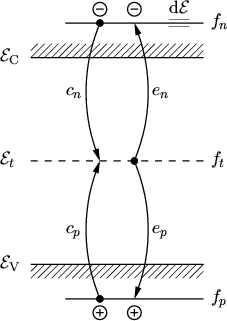Interaction among the partial systems electrons, holes, and traps is described by four partial processes (Fig. 2.5)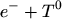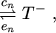(2.197)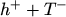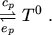(2.198):
Electron capture. An electron from the conduction band is trapped by an unoccupied defect which becomes occupied.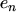:
Electron emission. An electron from an occupied trap moves to the conduction band. The trap becomes unoccupied.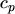:
Hole capture. An electron from an occupied trap moves to the valence band and neutralizes a hole. The trap becomes unoccupied.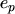:
Hole emission. An electron from the valence band is trapped by a defect, thus leaving a hole in the valence band and an occupied trap.
Here,,,are the respective rate constants. This description assumes acceptor-like traps which can exist in a neutral or a negatively charged state. Donor-like traps, which have a neutral and a positively charged state, lead however to exactly the same expression for the net recombination rate.

The generation- and recombination rates of electrons/holes within an energy interval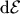are described by the law of mass action which states that the rates are proportional to the concentration of the involved reactants [43, p.54]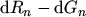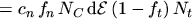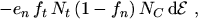(2.199)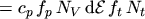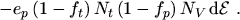(2.200)

The occupation probability of an energy level is given by the FERMI-DIRAC statistics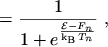(2.201)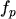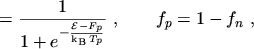(2.202)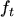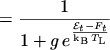(2.203)

with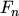,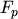, and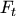being the respective quasi FERMI levels and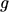the ground-state degeneracy of the trap [44, p.122] which is assumed to be 1 in the following.

Subsections

M. Gritsch: Numerical Modeling of Silicon-on-Insulator MOSFETs PDF